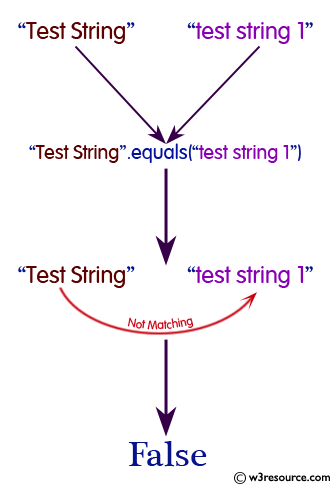# Java String: equals() Method

## public boolean equals(Object anObject)

The equals() method is used to compare a given string to the specified object. The result is true if and only if the argument is not null and is a String object that represents the same sequence of characters as this object.

Java Platform: Java SE 8

Syntax:

`equals(Object anObject)`

Parameters:

Name Description Type
anObject The object to compare this String against. Object

Return Value : true if the given object represents a String equivalent to this string, false otherwise.

Return Value Type: boolean

Pictorial presentation of Java String equals() MethodExample: Java String equals() Method

The following example shows the usage of java String() method.

``````public class Example {

public static void main(String[] args)
{
String columnist1 = "Walter Winchell";
String columnist2 = "John Gilbert";
String columnist3 = "Stephen Edwin King";

// Are any of the above Strings equal to one another?
boolean equals1 = columnist1.equals(columnist2);
boolean equals2 = columnist1.equals(columnist3);
System.out.println();
// Display the results of the equality checks.
System.out.println("\"" + columnist1 + "\" equals \"" +
columnist2 + "\"? " + equals1);
System.out.println("\"" + columnist1 + "\" equals \"" +
columnist3 + "\"? " + equals2);
System.out.println();
}
}
```
```

Output:

```"Walter Winchell" equals "John Gilbert"? false
"Walter Winchell" equals "Stephen Edwin King"? false
```

Java Code Editor:

Previous:endsWith Method
Next:equalsIgnoreCase Method

﻿